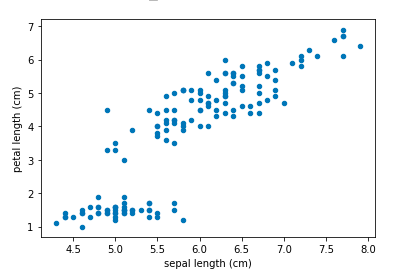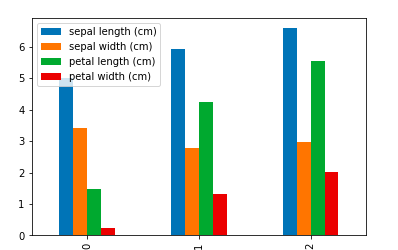Search
•Magdalena Konkiewicz

# 9 pandas visualizations techniques for effective data analysisImage by Mediamodifier from Pixabay

Introduction

In this article, I would like to present you with nine different visualization techniques that will help you analyze any data set. Most of these techniques require just one line of code. We all love simplicity, don’t we?

You will learn how to use:

line plot,

scatter plot,

area plot,

bar chart,

piechart,

histogram,

kernel density function,

boxplot,

and scatter matrix plot.

We will discuss all of the above visualization techniques, explore different ways of using them, and learn how to customize them to suit a data set.

Let’s get started.

Load data set and quick data exploration

For simplicity purposes, we will use the Iris data set that can be loaded from a scikit-learn library using the following code:

```
from sklearn.datasets import load_iris
import pandas as pd
df = pd.DataFrame(data['data'], columns=data['feature_names'])
df['species'] = data['target']

```As you can see we have a data set with five columns only. Let’s call info() function on the data frame for quick analysis:

```
df.info()

```As you can see there are only 150 entries, there are no missing values in any of the columns.

Additionally, we have learnt that the first four columns have float values whereas the last column allows integers only. In fact from the data set description we know that species column will take only three values each one representing one type of flower.

To confirm this you can call the unique() function on that column:

```
df.species.unique()
array([0, 1, 2])

```

Indeed species column take only three values: 0, 1, and 2.

Knowing this basic information about our data set we can proceed to visualizations. Note that if there were some missing values in the columns you should either drop them or fill them in. This is because some of the techniques we will discuss later on will not allow for missing values.

Line plot

We are going to start our visualizations with a simple line plot. Let’s just call it on the whole data frame.

```
df.plot()

```As you can see here it has plotted all the column values in different colours against the index value (x-axis). This is the default behaviour when we do not supply the x-axis parameter for the function.

As you can see this plot is not very useful. The line plot would be a good choice if the x-axis was a time series. Then we could probably see some trends in time within data.

In our case, we can only see that the data is ordered by species column (purple steps in the graph) and that some other columns have a moving average that follows that pattern (petal length especially that is marked in red).

Scatter plot

The next type of visualisation we are going to discover is a scatter plot. This a perfect type of plot to visualise correlations between two continuous variables. Let’s demonstrate it by plotting sepal length against sepal width.

```
df.plot.scatter(x='sepal length (cm)', y='sepal width (cm)')

```As you can see in order to produce this graph you need to specify x and y-axis for the plot by supplying its column names. This graph reveals that there is no strong correlation between the two variables. Let’s examine a different pair, sepal length and petal length:

```
df.plot.scatter(x='sepal length (cm)', y='petal length (cm)')

```In this case, we can see that when sepal length increases petal length increases as well (it is stronger for values of sepal length larger than 6 cm).

Area plot

Let’s create an area plot for the data frame. I will include all dimensions with centimetres in my plot but remove the species column as it will not make sense to include it in our case.

```
columns = ['sepal length (cm)', 'petal length (cm)', 'petal width (cm)', 'sepal width (cm)']
df[columns].plot.area()

```The measurements on this graph are stuck one on top of each other. Looking at this chart you can visually examine the ratio between each measurement that is included in the graph. You can see that all sizes have a growing trend towards the later entries.

Bar chart

This is the good type of a graph to include when showing averages or counts of entries. Let’s use it to compute averages for each dimension for each species in our data set. In order to do it, you will need to use a groupby() and mean() function. I am not going to explain how they work in detail here but you can check this article that explains these concepts.

```
df.groupby('species').mean().plot.bar()

```As you can see this is very straight forward to read. I can see that there are differences in average measurements for different species and different columns.

Piechart

You can use a pie chart in order to visualize the class count for your target variable. We will do it here for the Iris data set we are working on. Again we will need some helper functions. This time it is groupby() and count():

```
df.groupby('species').count().plot.pie(y='sepal length (cm)')

```As you can see we have perfect proportions for our classes as our data set consist of 50 entries for each class.

Note that we had to use y parameter here and set it to some column name. We have used sepal length column here but it could be any column as the counts are the same for all of them.

Histogram

This is a perfect visualization for any continuous variable. Let’s start with simple hist() function.

```
import matplotlib.pyplot as plt
df.hist()
plt.tight_layout()

```As you can see this produces a histogram for each numeric variable in the data set.

I had to add some extra lines of code in order to customize the graph. This is the first import line and the last line where I call tight_layout() function. If this is not added the labels and subgraph names may overlap and not be visible.

Kernel density function

In a similar way as a histogram you can use kernel density function:

```
df.plot.kde(subplots=True, figsize=(5,9))

```You can see that it gives similar results to the histogram.

We had to specify a figure size here as without it the graphs were squashed vertically too much. Also, we had set subplots parameter to True as by default all columns would be displayed on the same graph.

Boxplot

Another visualisation that should be used for numerical variables. Let’s create boxplots for all measurement columns (we are excluding species column as the box plot does not make sense for this categorical variable):

```
columns = ['sepal length (cm)', 'petal length (cm)', 'petal width (cm)', 'sepal width (cm)']
df[columns].plot.box()
plt.xticks(rotation='vertical')

```As you can see all boxplots are drawn on the same plot. This is fine in our case as we do not have too many variables to visualise.

Note that we had to rotate the x labels as without it the names of the labels were overlapping with each other.

Scatter matrix plot

This is one of my favourites visualisation technique from pandas as it allows you to do a quick analysis of all numerical values in the dataset and their correlations.

By default, it will produce scatterplots for all numeric pairs of variables and histograms for all numeric variables in the data frame:

```
from pandas.plotting import scatter_matrix
scatter_matrix(df, figsize=(10, 10))

```As you can the results is this beautiful set of plots that indeed can tell you a lot about the data set using only one line of code. I can spot some correlations between variables in this data set just by glancing at it.

The only additional parameter I had to set was figure size and this is because the plots were very small with a default size of the chart.

Summary

I hope you have enjoyed this short tutorial about different pandas visualisations techniques and that you will be able to apply this knowledge to a data set of your choice.

Happy graphing!
2,062 views1 comment